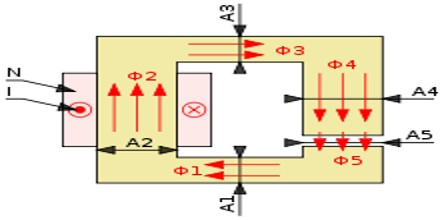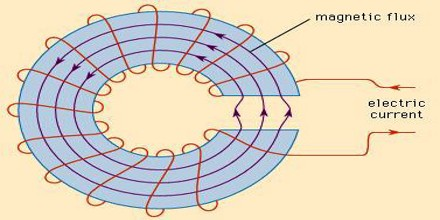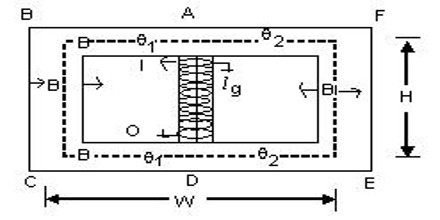Magnetic Circuit

Definition

A magnetic circuit is made up of magnetic materials having high permeability such as iron, soft steel, etc. It is the closed path followed by magnetic lines of forces is called magnetic circuit. In the magnetic circuit, magnetic flux or magnetic line of forces starts from a point and ends at the same point after completing its path. Flux is generated by magnets; it can be permanent magnet or electromagnets.The concept of a “magnetic circuit” exploits a one-to-one correspondence between the equations of the magnetic field in an unsaturated ferromagnetic material to that of an electrical circuit. Using this concept the magnetic fields of complex devices such as transformers can be quickly solved using the methods and techniques developed for electrical circuits.

Magnetic circuits are used in various devices like the electric motor, transformers, relays, generators galvanometer, etc. Consider a solenoid was having N turns wound on an iron core. The magnetic flux of ø Weber sets up in the core when the current of I ampere is passed through a solenoid.

Some examples of magnetic circuits are:

• Horseshoe magnet with iron keeper (low-reluctance circuit)
• Horseshoe magnet with no keeper (high-reluctance circuit)
• Electric motor (variable-reluctance circuit)

Structure and Functions of Magnetic Circuits

In a ring-shaped electromagnet with a small air gap, the magnetic field or flux is almost entirely confined to the metal core and the air gap, which together form the magnetic circuit. In an electric motor, the magnetic field is largely confined to the magnetic pole pieces, the rotor, the air gaps between the rotor and the pole pieces, and the metal frame. Each magnetic field line makes a complete unbroken loop. All the lines together constitute the total flux. If the flux is divided, so that part of it is confined to a portion of the device and part to another, the magnetic circuit is called parallel. If all the flux is confined to a single closed loop, as in a ring-shaped electromagnet, the circuit is called a series magnetic circuit.The magnetic flux is analogous to the electric current. The magnetomotive force, mmf, is analogous to the electromotive force and may be considered the factor that sets up the flux. The mmf is equivalent to a number of turns of wire carrying an electric current and has units of ampere-turns. If either the current through a coil (as in an electromagnet) or the number of turns of wire in the coil is increased, the mmf is greater; and if the rest of the magnetic circuit remains the same, the magnetic flux increases proportionally.

The magnetic flux through a magnetic component is proportional to the number of magnetic field lines that pass through the cross sectional area of that component. This is the net number, i.e. the number passing through in one direction, minus the number passing through in the other direction. The direction of the magnetic field vector B is by definition from the south to the North Pole of a magnet inside the magnet; outside the field lines go from north to south.

The flux through an element of area perpendicular to the direction of magnetic field is given by the product of the magnetic field and the area element. More generally, magnetic flux Φ is defined by a scalar product of the magnetic field and the area element vector.Application of Magnetic Circuit

The primary winding magnetic flux is able to excite / induce a magnetic flux density within the core material some 10000 times stronger than in air and it is this which allows the transformation of energy between the electric and magnetic fields.

In a step up transformer low voltage and high current is transformed to high voltage and low current and in so doing energy stored within the em fields are exchanged between the electric and magnetic fields but the total energy, and power, remains constant. A transformer allows the transfer and exchange of stored energy between the electric and magnetic fields it is the core material which makes this process significantly easier to due due to the property of the ferro-magnetic iron core material in aiding induction.

It is this exchange of energy between the electric and magnetic fields which allows us to exploit the properties of the conductors used in long transmission lines where such conductors are known to require very low internal electric field (volt drop). As such most of the energy is thus transferred within the electric field to allow much lower losses than if it same was done transferring the same amounts of energy stored in the magnetic field loss.